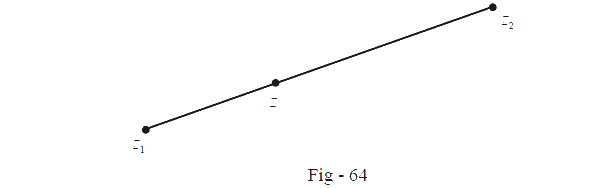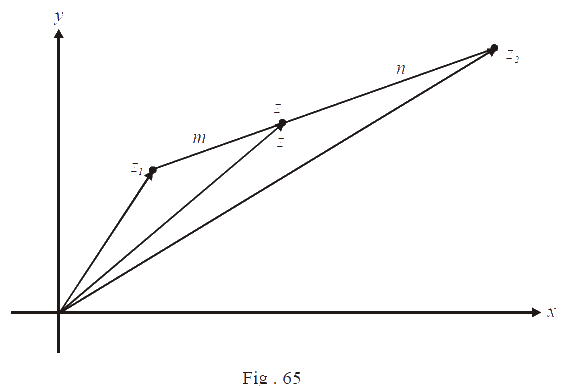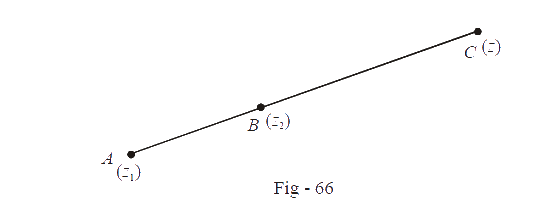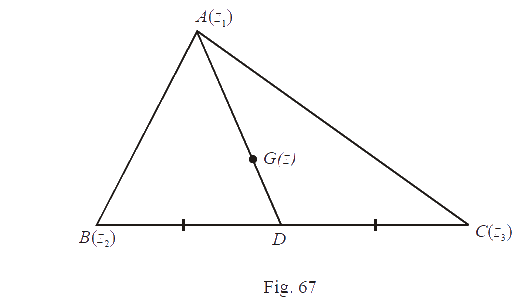# Distance And Section Formula With Complex Numbers

Go back to  'Complex Numbers'

In this section, we’ll put complex numbers to more geometrical use. We will write down equations of straight lines and circles, among other things, in the complex plane. Readers who are familiar with co-ordinate geometry an vectors will find this section very easy. Before proceeding, pay attention to the following facts necessary to do geometry with complex numbers.

* $$|{z_1} - {z_2}|$$ should be interpreted as the distance between the points $$z{_1}\,{\rm{and\,}}z{_2}$$ , or equivalently, the length of the vector $$z{_1}{\rm{ }}-z{_2}$$ .

*You must know the difference between the point $$z$$ and the vector $$z.$$ Suppose we talk about the point $$1 + i.$$ This has a fixed location in the plane, as you know, in the first quadrant. There’s also the vector $$1 + i,$$ which has an $$x$$-component of 1 and a $$y$$-component of 1, but this vector is not fixed. It is free vector. We can move the vector $$1 + i$$ anywhere around the plane as we wish, as long as we don’t change its magnitude and direction

*If $$z$$ is purely real, then $$z = \bar z$$ . If $$z$$ is purely imaginary, then $$z + \bar z = 0.$$

*Suppose $${z_1}\;and\;{\rm{ }}{z_2}$$  are fixed in the plane.We want to find a point $$z$$ on the line segment joining $${z_1}\,{\rm{ and\;}}{z_2}$$ such that this point divides the line segment in the ratio $$m : n. z$$ will be given by

$z = \frac{{m{z_2} + n{z_1}}}{{m + n}}$

How?

Lets $${z_1},{z_2}$$ consider and $$z$$ with respect to a reference axisNow, from the figure, it should be clear that

\begin{align} z - {z_1} &= \frac{m}{{m + n}}({z_2-z_1})\,\,\,\,\,\,\,\,\,\,\,\,\,\left( \text{verify this} \right)\\\\ \Rightarrow \quad z &= \frac{{m{z_2} + n{z_1}}}{{m + n}} \end{align}

Such a point $$z$$ is said to divide the line segment joining  $${z_1}\;{\rm{and\; }}{z_2}$$  internally in the ratio $$m:n.$$  We could also have an external division as follows.Here, $$z$$ divides the line segment joining  $${z_1}\;{\rm{ and\;}}{z_2}$$   externally in the ratio $$m : n,$$ i.e.,

$\frac{{AC}}{{CB}} = \frac{m}{n}$

Verify that $$z$$ in this case is given by

$z = \frac{{m{z_2} - n{z_1}}}{{m - n}}$

Now we apply our knowledge of complex numbers along with these facts to actual geometric problems, in the following examples.

Example - 31

(a) Write the equation of a circle of radius 1 centred at $$1 + i.$$

(b)What relation should $$z$$ satisfy so that it is closer to 1 than to $$i$$?

Solution:   (a)   Let $$z$$ be a variable complex number representing the required locus (circle). We want that the distance of $$z$$ from $$1 + i$$ must always equal 1.

$\Rightarrow |z - (1 + i)|\, = 1$

(b) The required condition can be easily translated into a mathematical form:

(Distance of $$z$$ from 1)    <     (Distance of $$z$$ from $$i$$)

$\Rightarrow |z - 1|\,\,\,\, < \,\,\,\,\,|z - i|$

Example - 32

If $${z_1}{\rm{ }},{z_2}\;{\rm{and\; }}{\rm{ }}{z_3}$$  represent the vertices of an arbitrary triangle, find its centroid.

Solution: Let $$ABC$$ be the triangle, and let $$G$$ be its centroid. Let $$D$$ be the mid point of $$BC.$$Since $$D$$ is the mid-point of $$BC$$ ($$D$$ divides $$BC$$ in the ratio $$1 : 1$$), $$D$$ is given by \begin{align}&\frac{{{z_2} + {z_3}}}{2} \end{align}. From plane geometry, we also know that the centroid divides any median in the ratio $$2: 1.$$ Thus,

$$AG : GD = 2 : 1$$

This implies that $$G$$ is given by

\begin{align}& z = \frac{{2\left( {\frac{{{z_2} + {z_3}}}{2}} \right) + 1\left( {{z_1}} \right)}}{{2 + 1}}\\ \,\,\,\,\,\,\,\,\,\,\,&\;\;=\frac{{{z_1} + {z_2} + {z_3}}}{3}\\ \end{align}

Thus, the centroid is given by \begin{align}&\frac{{{z_1} + {z_2} + {z_3}}}{3}.\end{align}

Learn from the best math teachers and top your exams

• Live one on one classroom and doubt clearing
• Practice worksheets in and after class for conceptual clarity
• Personalized curriculum to keep up with school